2019/08/08 14:54

【宋】张先

# 1、月来

``<circle id="whitemoon" cx="420" cy="160" r="60" fill="white" />``

``````<filter id="blur-moon">
<feGaussianBlur in="SourceGraphic" stdDeviation="3" />
</filter>``````

``````<use xlink:href="#whitemoon" />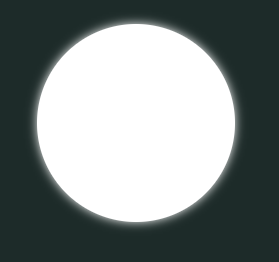``````<circle id="greymoon" cx="420" cy="170" r="45" fill="grey" fill-opacity="0.15"
filter="url(#filter-greymoon)" />
<filter id="filter-greymoon" >
<feTurbulence type="fractalNoise" baseFrequency="0.03" numOctaves="9" seed="3" />
<feDisplacementMap in="SourceGraphic" scale="120" />
</filter>``````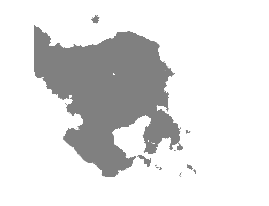``<filter id="filter-greymoon" height="250%" width="250%" x="-100%" y="-100%">``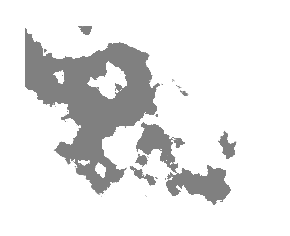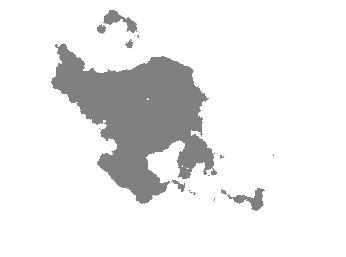``````<circle id="whitemoon" cx="420" cy="160" r="60" fill="white" />
<circle id="greymoon" cx="420" cy="170" r="30" fill="grey" fill-opacity="0.25"
filter="url(#filter-greymoon)" />
<filter id="filter-greymoon" width="350%" height="250%" x="-100%" y="-100%">
<feTurbulence type="fractalNoise" baseFrequency="0.03" numOctaves="9" seed="3" />
<feDisplacementMap in="SourceGraphic" scale="120" />
</filter>
<g id="moon">
</g>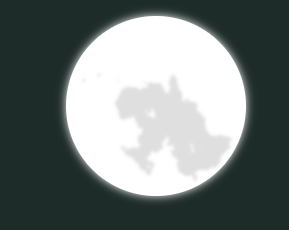# 2、云破

``````<defs>
<ellipse id="cloudback" cx="260" cy="230" rx="280" ry="70" fill="white" fill-opacity="0.9"
filter="url(#blur-cloud)" />
<ellipse id="cloudmid" cx="260" cy="240" rx="220" ry="55" fill="#9EA8B3" fill-opacity="0.5"
filter="url(#blur-cloud)" />
<ellipse id="cloudfront" cx="260" cy="260" rx="170" ry="30" fill="black" fill-opacity="0.2"
filter="url(#blur-cloud)" />
<filter id="blur-cloud" width="350%" height="250%" x="-100%" y="-100%">
<feGaussianBlur in="SourceGraphic" stdDeviation="15" />
</filter>
<filter id="filter-back" width="350%" height="250%" x="-100%" y="-100%">
<feTurbulence type="fractalNoise" baseFrequency="0.012" numOctaves="4" seed="0" />
<feDisplacementMap in="SourceGraphic" scale="170" />
</filter>
<filter id="filter-mid" width="350%" height="250%" x="-100%" y="-100%">
<feTurbulence type="fractalNoise" baseFrequency="0.012" numOctaves="4" seed="0" />
<feDisplacementMap in="SourceGraphic" scale="150" />
</filter>
<filter id="filter-front" width="350%" height="250%" x="-100%" y="-100%">
<feTurbulence type="fractalNoise" baseFrequency="0.012" numOctaves="4" seed="0" />
<feDisplacementMap in="SourceGraphic" scale="100" />
</filter>
<g id="cloud">
</g>
</defs>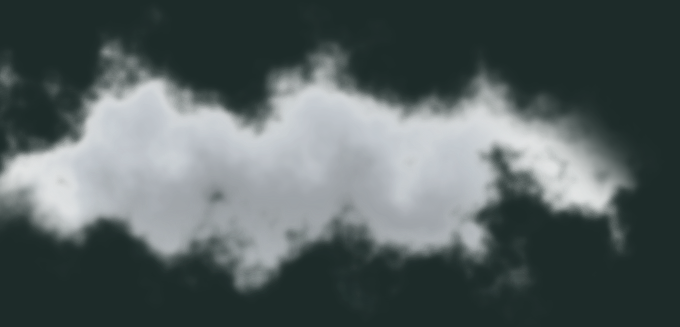# 3、花弄影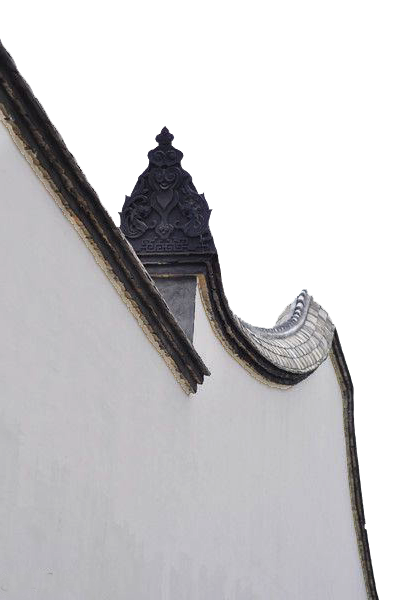``<image xlink:href="yuanqiang1.png" x="0" y="300" width="400"/>``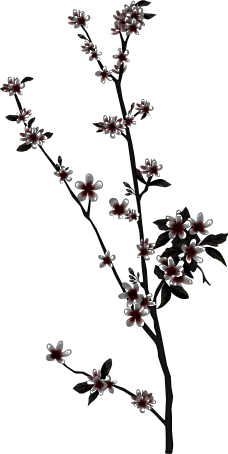``<image xlink:href="huazhi1.png" x="200"y="690" width="170"></image>``

``````<filter id="huazhishadow" x="0" y="0" width="200%" height="200%">
<feOffset result="offOut" in="SourceAlpha" dx="210" dy="0" />
<feGaussianBlur result="blurOut" in="offOut" stdDeviation="1" />
<feBlend in="SourceGraphic" in2="blurOut" mode="darken" />
</filter>``````

feoffset：该输入图像作为一个整体，在属性`dx`和属性`dy`的值指定了它的偏移量。说白了就是把原图在离开dx和dy的距离外复制一个一样的图像，活脱脱的拷贝魔法，这就是filter界的旗木卡卡西啊。

feGaussianBlur：老朋友，干模糊化的，略

feBlend：把两个对象组合在一起，使它们受特定的混合模式控制。这类似于图像编辑软件中混合两个图层。它是个粘合剂，把上面两个filter的效果合在一起，并且用mode来控制最后的效果。mode的效果有很多种，我们这边需要的是影子，所以选择了darken，具体的mode说明，请看这里

``<image xlink:href="huazhi1.png" x="0" y="600" width="170" filter="url(#huazhishadow)" />``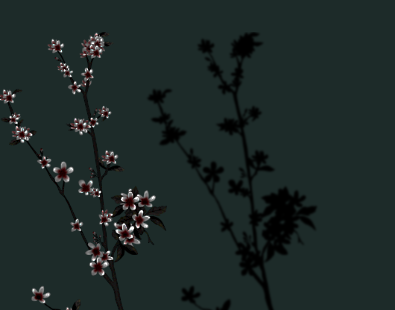-------------------------------------------------------我是分割线----------------------------------------------

``<image xlink:href="huazhi1.png" x="-630" y="720" width="170" filter="url(#huazhishadow)">``

``<image xlink:href="huazhi1.png" x="-630" y="720" width="170" filter="url(#huazhishadow)" transform="skewX(29)"/>``

transform和上面这些filter不同，是SVG元素的属性。作用是设置svg元素的变形，包括位移`translate`, 旋转`rotate`, 缩放`scale`, 斜切`skew。今天我们用到的就是斜切（skew）。具体的大家可以去看这篇文章，讲的很详细。最后的花影的效果如下：`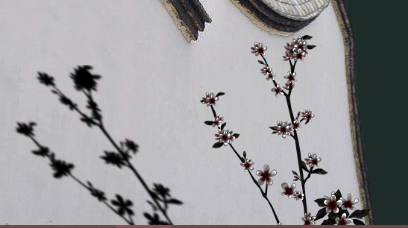# 4、成品

``````<image xlink:href="yuanqiang1.png" x="0" y="300" width="400"/>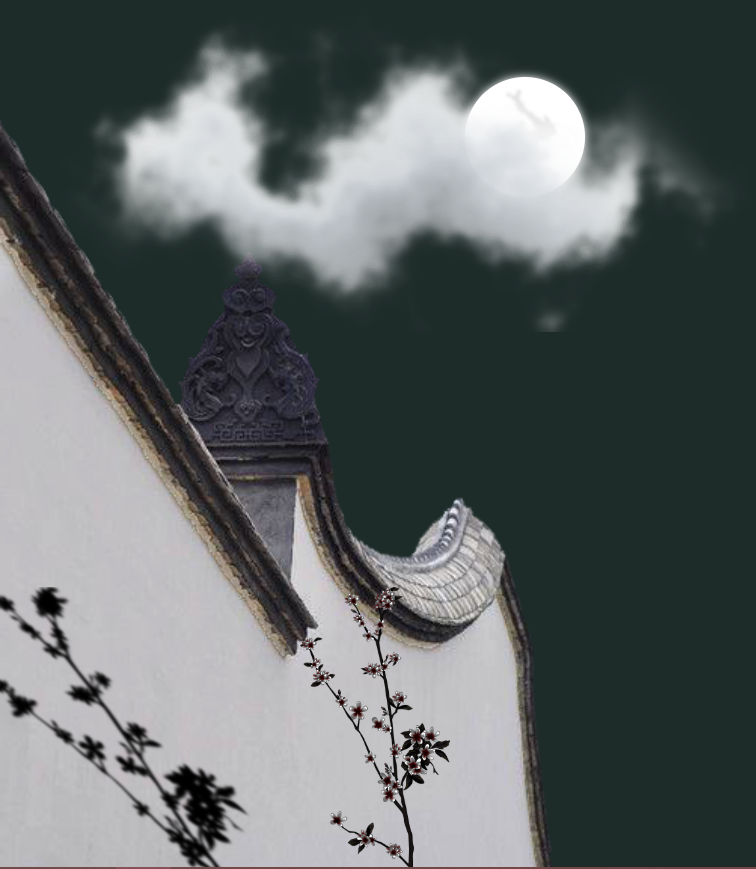1
4 收藏

### 作者的其它热门文章0 评论
4 收藏
1